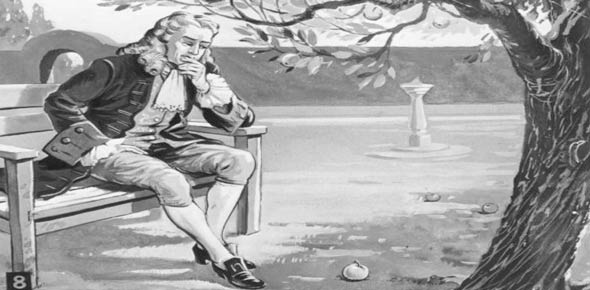# Newton's Law Quiz: How Much You Know?

10 Questions | Total Attempts: 4638SettingsIsaac Newton was a renowned and revered physicist and mathematician who evolved the law of gravitation. He developed the three laws of motion. The following quiz will test your conceptual understanding and test your knowledge of Newton's three laws of motion. Let's try it out!

• 1.
If the weight of a hot air balloon is 3000N and the lift force provided is 3300N, what is the net force acting on the balloon?
• A.

3300N up

• B.

3000N down

• C.

300N up

• D.

300N down

• 2.
Weight is a measure of?
• A.

Inertia

• B.

The force of gravity acting on an object

• C.

The normal force acting on an object

• D.

The frictional force acting on an object

• 3.
Which of Newton's Three Laws does the following statement satisfy? The relationship between an object's mass (m), its acceleration (a), and the applied force F is F=ma. Acceleration and force are vectors. This law requires that the direction of the acceleration vector is in the same direction as the force vector.
• A.

Newton's First Law

• B.

Newton's Second Law

• C.

Newton's Third Law

• D.

All of the above

• 4.
Which of Newton's Three Laws does the following statement satisfy? For every action, there is an equal and opposite reaction.
• A.

Newton's First Law

• B.

Newton's Second Law

• C.

Newton's Third Law

• D.

All of the above

• 5.
Which of Newton's Three Laws does the following statement satisfy? Every object in a state of uniform motion tends to remain in that state of motion unless an external force is applied to it.
• A.

Newton's First Law

• B.

Newton's Second Law

• C.

Newton's Third Law

• D.

All of the above

• 6.
Which law states the need to wear seatbelts?
• A.

Newton's First Law

• B.

Newton's Second Law

• C.

Newton's Third Law

• D.

None of the above

• 7.
Which law helps to define "inertia?"
• A.

1st Law

• B.

2nd Law

• C.

3rd Law

• 8.
What does Newton's 2nd Law say?
• A.

A object will always move at a constant velocity unless acted upon by an unbalanced force.

• B.

A object will always move with a changing velocity while it is acted upon by an unbalanced force

• 9.
Which law of motion says that the harder you kick a ball, the farther it will go?
• A.

First Law of Motion

• B.

Second Law of Motion

• C.

Third Law of Motion

• 10.
Which law of motion says that a kicked ball would fly forever if it wasn't for forces like air friction and gravity?
• A.

First Law of Motion

• B.

Second Law of Motion

• C.

Third Law of Motion

Related TopicsBack to top